StatLect

Gauss Markov theorem

The Gauss Markov theorem says that, under certain conditions, the ordinary least squares (OLS) estimator of the coefficients of a linear regression model is the best linear unbiased estimator (BLUE), that is, the estimator that has the smallest variance among those that are unbiased and linear in the observed output variables.Assumptions

The regression model iswhere:

•is anvector of observations of the output variable (is the sample size);

•is anmatrix of inputs (is the number of inputs for each observation);

•is avector of regression coefficients;

•is anvector of errors.

The OLS estimator ofisWe assume that:

1.has full-rank (as a consequence,is invertible, andis well-defined);

2.;

3., whereis theidentity matrix andis a positive constant.

OLS is linear and unbiased

First of all, note thatis linear in. In fact,is the product between thematrixand, and matrix multiplication is a linear operation.

It can easily be proved thatis unbiased, both conditional on, and unconditionally, that is,Proof

We can use the definition ofto re-write the OLS estimator as follows:When we condition on, we can treatas a constant matrix. Therefore, the conditional expectation ofis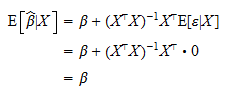The Law of Iterated Expectations implies thatWhat it means to be best

Now that we have shown that the OLS estimator is linear and unbiased, we need to prove that it is also the best linear unbiased estimator.

What exactly do we mean by best?

Whenis a scalar (i.e., there is only one regressor), we considerto be the best among those we are considering (i.e., among all the linear unbiased estimators) if and only if it has the smallest possible variance, that is, if its deviations from the true valuetend to be the smallest on average. Thus,is the best linear unbiased estimator (BLUE) if and only iffor any other linear unbiased estimator.

Since we often deal with more than one regressor, we have to extend this definition to a multivariate context. We do this by requiring thatfor anyconstant vector, any other linear unbiased estimator.

In other words, OLS is BLUE if and only if any linear combination of the regression coefficients is estimated more precisely by OLS than by any other linear unbiased estimator.

Condition (1) is satisfied if and only ifis a positive semi-definite matrix.

Proof

We can write condition (1) asor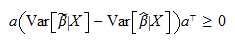But the latter inequality is true if and only ifis positive-semidefinite (by the very definition of positive-semidefinite matrix).

In the next two sections we will derive(the covariance matrix of the OLS estimator), and then we will prove that (2) is positive-semidefinite, so that OLS is BLUE.

The covariance matrix of the OLS estimator

The conditional covariance matrix of the OLS estimator isProof

We have already proved (see above) that the OLS estimator can be written asTherefore, its conditional variance isOLS is BLUE

Since we are considering the set of linear estimators, we can write any estimator in this set aswhereis amatrix.

Furthermore, if we definethen we can writeIt is possible to prove thatifis unbiased.

Proof

We have that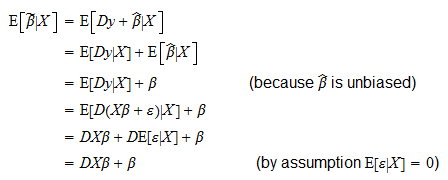As a consequence,is always equal toonly if.

By using this result, we can also prove that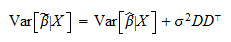Proof

The proof is as follows:where in steps,andwe have used the fact that.

As a consequence,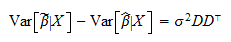is positive semi-definite becauseis positive semi-definite. This is true for any unbiased linear estimator. Therefore, the OLS estimator is BLUE.

The book

Most of the learning materials found on this website are now available in a traditional textbook format.

Glossary entries
Share